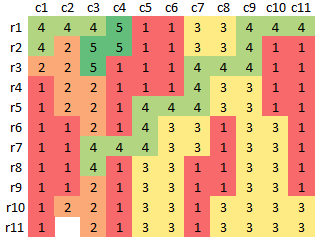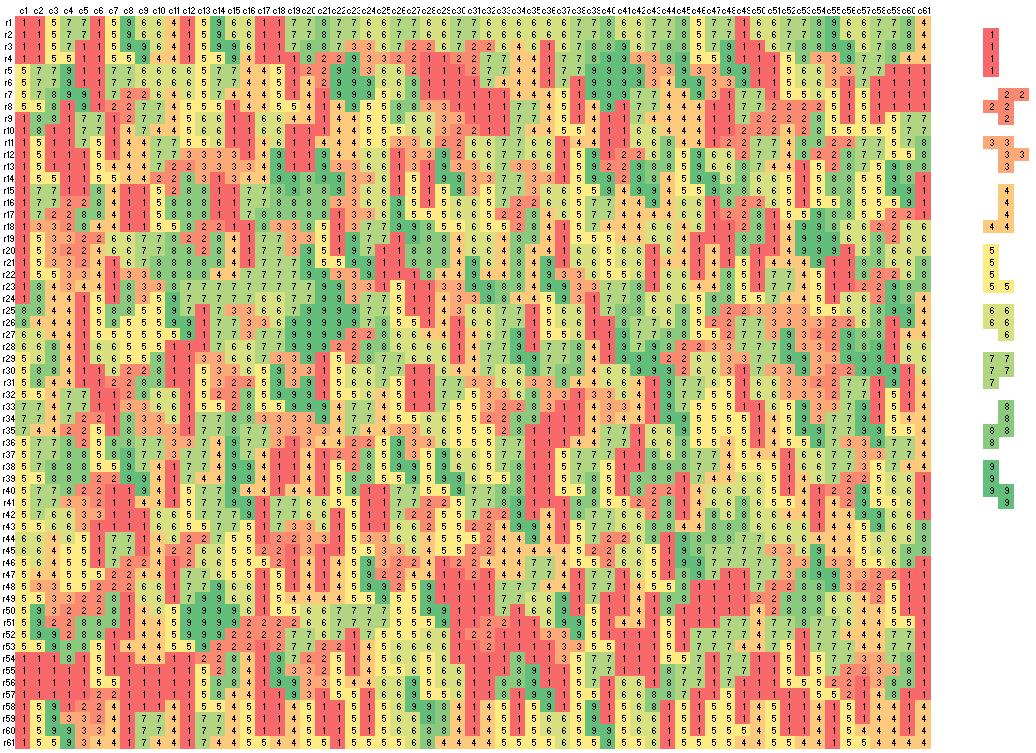Wednesday, December 27, 2017

Filling rectangles with polyominos

I know about dominos, but polyominos  are new for me. In  a problem is posted: fill a rectangle (or square) with a number of different polyominos. No overlap or rotation allowed. I assume there is no limitation in the supply of polyominos.

For example, consider a $$11 \times 11$$ grid, and choose from the following building blocks:Five different polyominos

We can try to fill our grid, but we will not always succeed:Fail to cover complete grid

I solved this as a MIP as follows. My decision variables are:

$x_{i,j,k} = \begin{cases} 1 & \text{if we place polyomino k at location (i,j)}\\ 0 & \text{otherwise} \end{cases}$

I used as rule that the left-upper corner of each polynomino is its anchor. I.e. in the picture above we have $$x_{1,1,4} = 1$$, $$x_{2,1,2}=1$$, $$x_{1,3,5}=1$$ etc.

To formulate a non-overlap constraint I populated a set $$cover_{i,j,k,i',j'}$$, with elements that exist  if cell $$(i',j')$$ is covered when we place polyomino $$k$$ in cell $$(i,j)$$. To require each cell $$(i',j')$$ is covered we can say:

$\forall i',j': \sum_{i,j,k|cover_{i,j,k,i',j'}} x_{i,j,k} = 1$

This constraint is infeasible if we cannot cover each cell $$(i',j')$$ exactly once. In order to make sure we can show a meaningful solution when we cannot cover each cell, we formulate the following optimization model:

\begin{align} \max\>&\sum_{i,j} y_{i,j}\\&y_{i',j'} = \sum_{i,j,k|cover_{i,j,k,i',j'}} x_{i,j,k}\\&x_{i,j,k}\in \{0,1\}\\&y_{i,j} \in \{0,1\}\end{align}

Here $$y_{i,j}=1$$ indicates cell $$(i,j)$$ is covered exactly once, and $$y_{i,j}=0$$ says the cell is not covered.

With a little bit of effort we can produce the following:61 x 61 board with 9 different polyominos
A SAT solver may be better suited for this , but MIP works for all but very large instances.

References

1. Polyomino, https://en.wikipedia.org/wiki/Polyomino
2. 2D bin packing on a grid, https://stackoverflow.com/questions/47918792/2d-bin-packing-on-a-grid